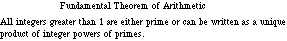index: click on a letter A B C D E F G H I J K L M N O P Q R S T U V W X Y Z A to Z index index: subject areas numbers & symbols sets, logic, proofs geometry algebra trigonometry advanced algebra & pre-calculus calculus advanced topics probability & statistics real world applications multimedia entrieswww.mathwords.com about mathwords website feedback

 Fundamental Theorem of Arithmetic The assertion that prime factorizations are unique. That is, if you have found a prime factorization for a positive integer then you have found the only such factorization. There is no different factorization lurking out there somewhere.See also Prime number